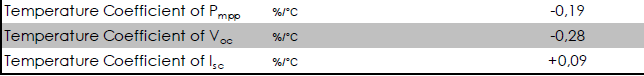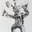Question-and-Answer Resource for the Building Energy Modeling Community
Get s tarted with the Help page

# Is A/K or V/K same as %/C on Temperature Coefficient?I m trying to model BiPV panels with E+. I ve been using the PhotovoltaicPerformance:Equivalent One-Diode model. As i was filling the variables i noticed that Temperature Coefficient of Isc and Voc units are A/K and V/K. In the product manual that i m using both variables have %/C as units. Is it the same thing or do i have to convert the units? Thank you in advanceedit retag close merge delete

Sort by » oldest newest most voted/K and /C are equivalent, but not %. Take the mfr %/C for Voc and multiply it by the value for Open Circuit Voltage [V] to get V/K. Likewise, multiply the %/C for Isc by the value for Short Circuit Current[A].

more